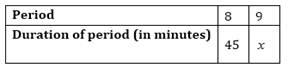# A school has 8 periods a day each of 45 minutes duration. How long would each period be, if the school has 9 periods a day, assuming the number of school hours to be the same?

Solution

Let the duration of each period be x.Here the number of periods and the duration of periods are inversly proportional

8/9 = x/45

8×45 = 9x

x = 40

Hence, duration of each period would be 40 minutes.